# Creating multiple subplots using plt.subplots¶

pyplot.subplots creates a figure and a grid of subplots with a single call, while providing reasonable control over how the individual plots are created. For more advanced use cases you can use GridSpec for a more general subplot layout or Figure.add_subplot for adding subplots at arbitrary locations within the figure.

import matplotlib.pyplot as plt
import numpy as np

# Some example data to display
x = np.linspace(0, 2 * np.pi, 400)
y = np.sin(x ** 2)


## A figure with just one subplot¶

subplots() without arguments returns a Figure and a single Axes.

This is actually the simplest and recommended way of creating a single Figure and Axes.

fig, ax = plt.subplots()
ax.plot(x, y)
ax.set_title('A single plot')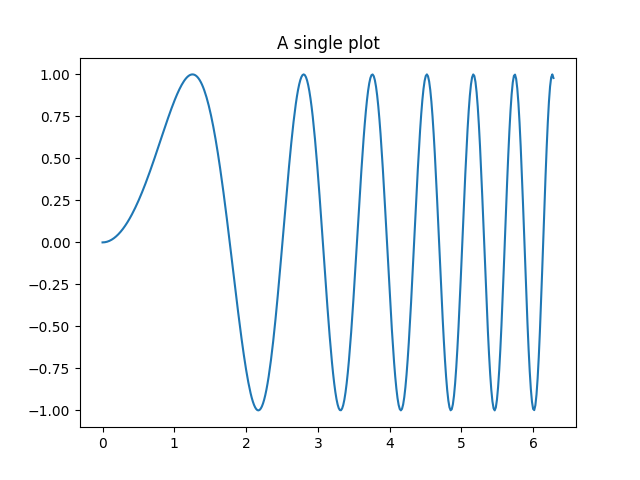Out:

Text(0.5, 1.0, 'A single plot')


## Stacking subplots in one direction¶

The first two optional arguments of pyplot.subplots define the number of rows and columns of the subplot grid.

When stacking in one direction only, the returned axs is a 1D numpy array containing the list of created Axes.

fig, axs = plt.subplots(2)
fig.suptitle('Vertically stacked subplots')
axs.plot(x, y)
axs.plot(x, -y)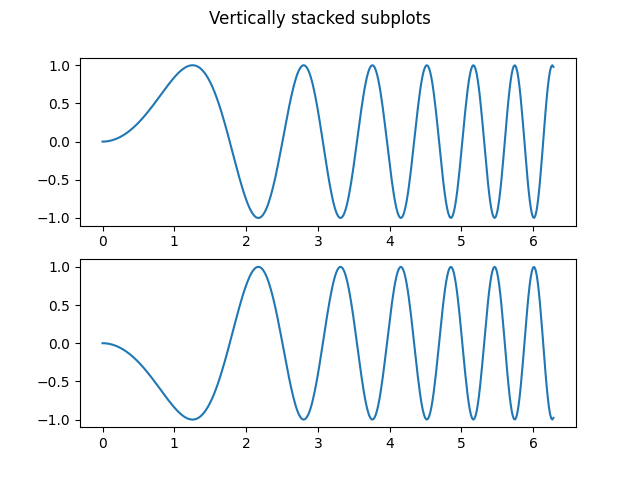Out:

[<matplotlib.lines.Line2D object at 0x7fcbe3930a30>]


If you are creating just a few Axes, it's handy to unpack them immediately to dedicated variables for each Axes. That way, we can use ax1 instead of the more verbose axs.

fig, (ax1, ax2) = plt.subplots(2)
fig.suptitle('Vertically stacked subplots')
ax1.plot(x, y)
ax2.plot(x, -y)Out:

[<matplotlib.lines.Line2D object at 0x7fcbe3bcda00>]


To obtain side-by-side subplots, pass parameters 1, 2 for one row and two columns.

fig, (ax1, ax2) = plt.subplots(1, 2)
fig.suptitle('Horizontally stacked subplots')
ax1.plot(x, y)
ax2.plot(x, -y)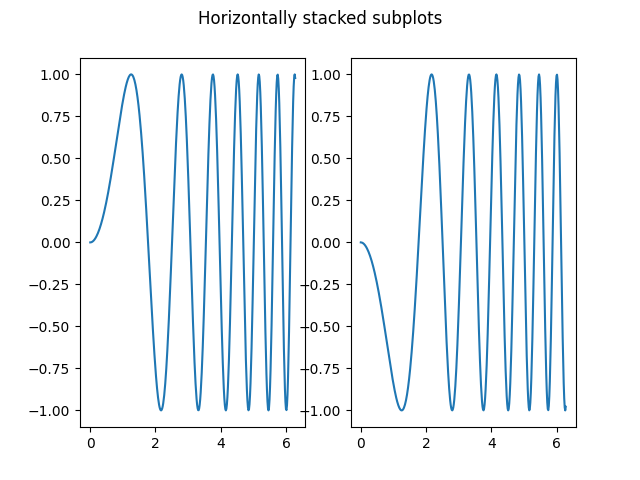Out:

[<matplotlib.lines.Line2D object at 0x7fcbe3f9bd00>]


## Stacking subplots in two directions¶

When stacking in two directions, the returned axs is a 2D NumPy array.

If you have to set parameters for each subplot it's handy to iterate over all subplots in a 2D grid using for ax in axs.flat:.

fig, axs = plt.subplots(2, 2)
axs[0, 0].plot(x, y)
axs[0, 0].set_title('Axis [0, 0]')
axs[0, 1].plot(x, y, 'tab:orange')
axs[0, 1].set_title('Axis [0, 1]')
axs[1, 0].plot(x, -y, 'tab:green')
axs[1, 0].set_title('Axis [1, 0]')
axs[1, 1].plot(x, -y, 'tab:red')
axs[1, 1].set_title('Axis [1, 1]')

for ax in axs.flat:
ax.set(xlabel='x-label', ylabel='y-label')

# Hide x labels and tick labels for top plots and y ticks for right plots.
for ax in axs.flat:
ax.label_outer()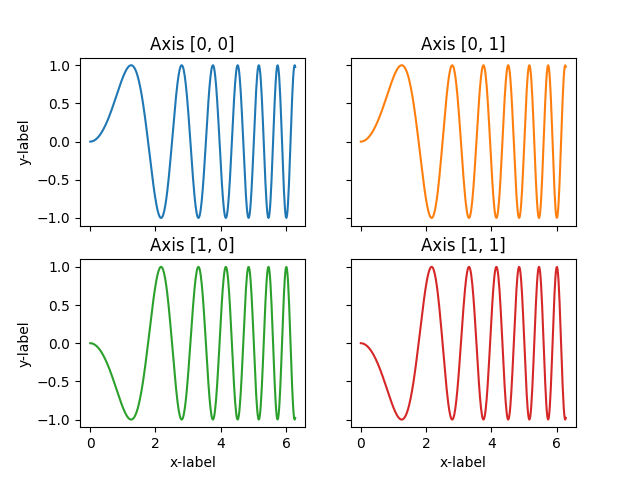You can use tuple-unpacking also in 2D to assign all subplots to dedicated variables:

fig, ((ax1, ax2), (ax3, ax4)) = plt.subplots(2, 2)
fig.suptitle('Sharing x per column, y per row')
ax1.plot(x, y)
ax2.plot(x, y**2, 'tab:orange')
ax3.plot(x, -y, 'tab:green')
ax4.plot(x, -y**2, 'tab:red')

for ax in fig.get_axes():
ax.label_outer()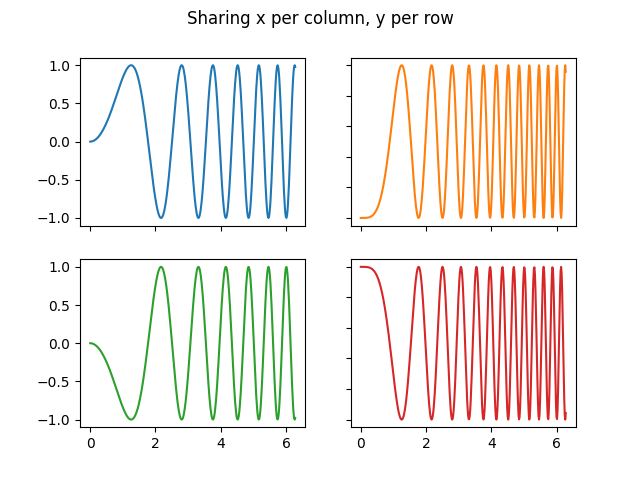## Sharing axes¶

By default, each Axes is scaled individually. Thus, if the ranges are different the tick values of the subplots do not align.

fig, (ax1, ax2) = plt.subplots(2)
fig.suptitle('Axes values are scaled individually by default')
ax1.plot(x, y)
ax2.plot(x + 1, -y)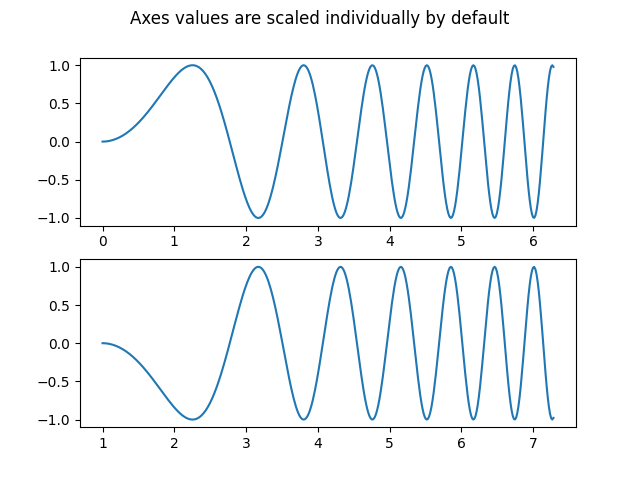Out:

[<matplotlib.lines.Line2D object at 0x7fcbe3a644c0>]


You can use sharex or sharey to align the horizontal or vertical axis.

fig, (ax1, ax2) = plt.subplots(2, sharex=True)
fig.suptitle('Aligning x-axis using sharex')
ax1.plot(x, y)
ax2.plot(x + 1, -y)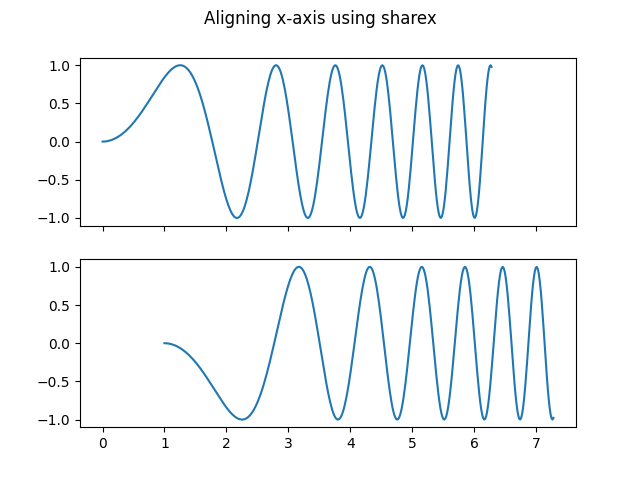Out:

[<matplotlib.lines.Line2D object at 0x7fcbe3da15e0>]


Setting sharex or sharey to True enables global sharing across the whole grid, i.e. also the y-axes of vertically stacked subplots have the same scale when using sharey=True.

fig, axs = plt.subplots(3, sharex=True, sharey=True)
fig.suptitle('Sharing both axes')
axs.plot(x, y ** 2)
axs.plot(x, 0.3 * y, 'o')
axs.plot(x, y, '+')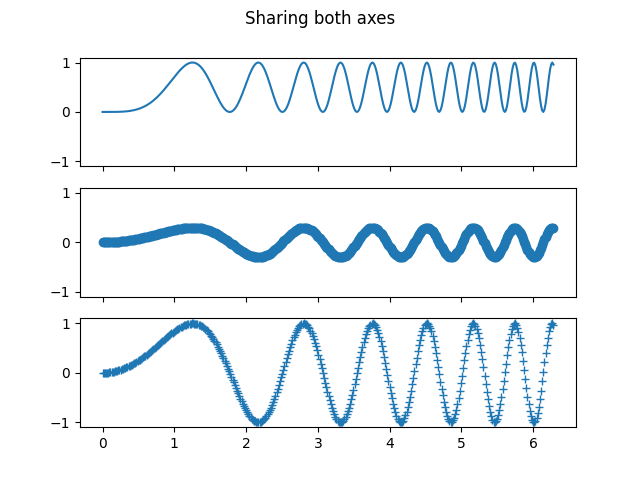Out:

[<matplotlib.lines.Line2D object at 0x7fcbe3ec2e80>]


For subplots that are sharing axes one set of tick labels is enough. Tick labels of inner Axes are automatically removed by sharex and sharey. Still there remains an unused empty space between the subplots.

To precisely control the positioning of the subplots, one can explicitly create a GridSpec with add_gridspec, and then call its subplots method. For example, we can reduce the height between vertical subplots using add_gridspec(hspace=0).

label_outer is a handy method to remove labels and ticks from subplots that are not at the edge of the grid.

fig = plt.figure()
axs = gs.subplots(sharex=True, sharey=True)
fig.suptitle('Sharing both axes')
axs.plot(x, y ** 2)
axs.plot(x, 0.3 * y, 'o')
axs.plot(x, y, '+')

# Hide x labels and tick labels for all but bottom plot.
for ax in axs:
ax.label_outer()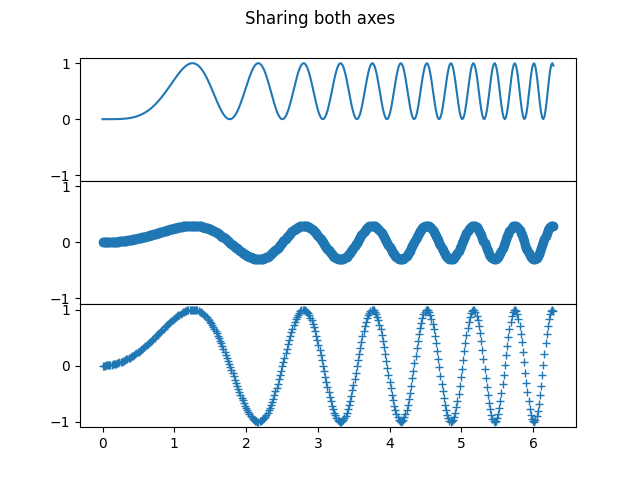Apart from True and False, both sharex and sharey accept the values 'row' and 'col' to share the values only per row or column.

fig = plt.figure()
gs = fig.add_gridspec(2, 2, hspace=0, wspace=0)
(ax1, ax2), (ax3, ax4) = gs.subplots(sharex='col', sharey='row')
fig.suptitle('Sharing x per column, y per row')
ax1.plot(x, y)
ax2.plot(x, y**2, 'tab:orange')
ax3.plot(x + 1, -y, 'tab:green')
ax4.plot(x + 2, -y**2, 'tab:red')

for ax in axs.flat:
ax.label_outer()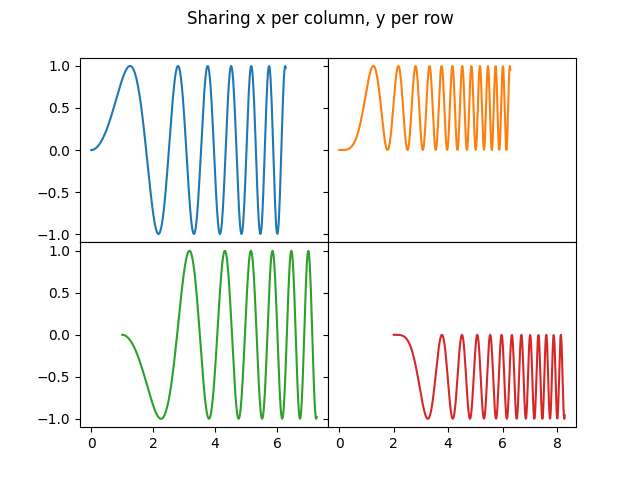If you want a more complex sharing structure, you can first create the grid of axes with no sharing, and then call axes.Axes.sharex or axes.Axes.sharey to add sharing info a posteriori.

fig, axs = plt.subplots(2, 2)
axs[0, 0].plot(x, y)
axs[0, 0].set_title("main")
axs[1, 0].plot(x, y**2)
axs[1, 0].set_title("shares x with main")
axs[1, 0].sharex(axs[0, 0])
axs[0, 1].plot(x + 1, y + 1)
axs[0, 1].set_title("unrelated")
axs[1, 1].plot(x + 2, y + 2)
axs[1, 1].set_title("also unrelated")
fig.tight_layout()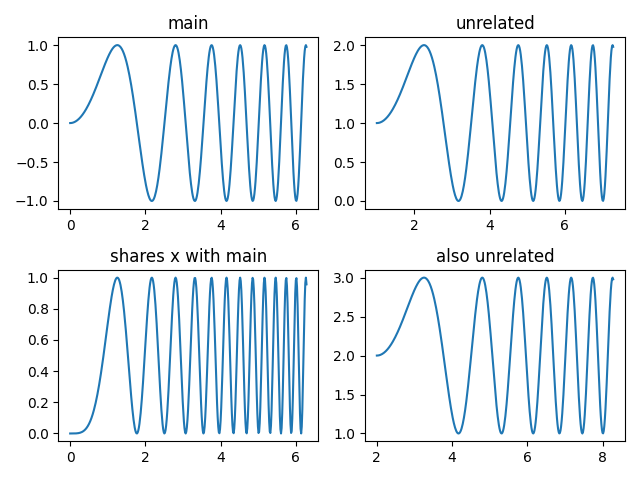## Polar axes¶

The parameter subplot_kw of pyplot.subplots controls the subplot properties (see also Figure.add_subplot). In particular, this can be used to create a grid of polar Axes.

fig, (ax1, ax2) = plt.subplots(1, 2, subplot_kw=dict(projection='polar'))
ax1.plot(x, y)
ax2.plot(x, y ** 2)

plt.show()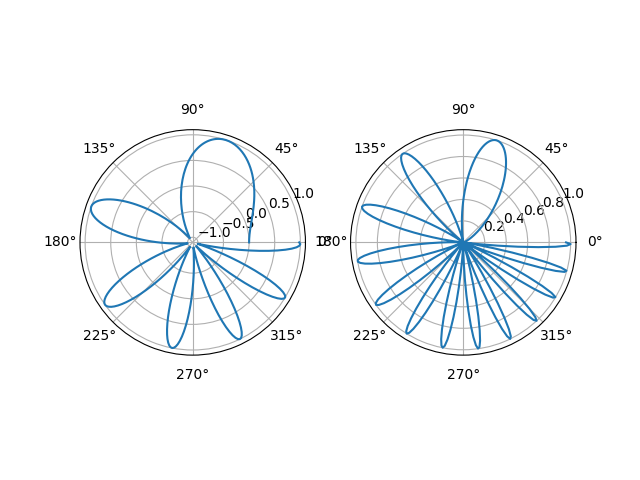Total running time of the script: ( 0 minutes 6.711 seconds)

Keywords: matplotlib code example, codex, python plot, pyplot Gallery generated by Sphinx-Gallery